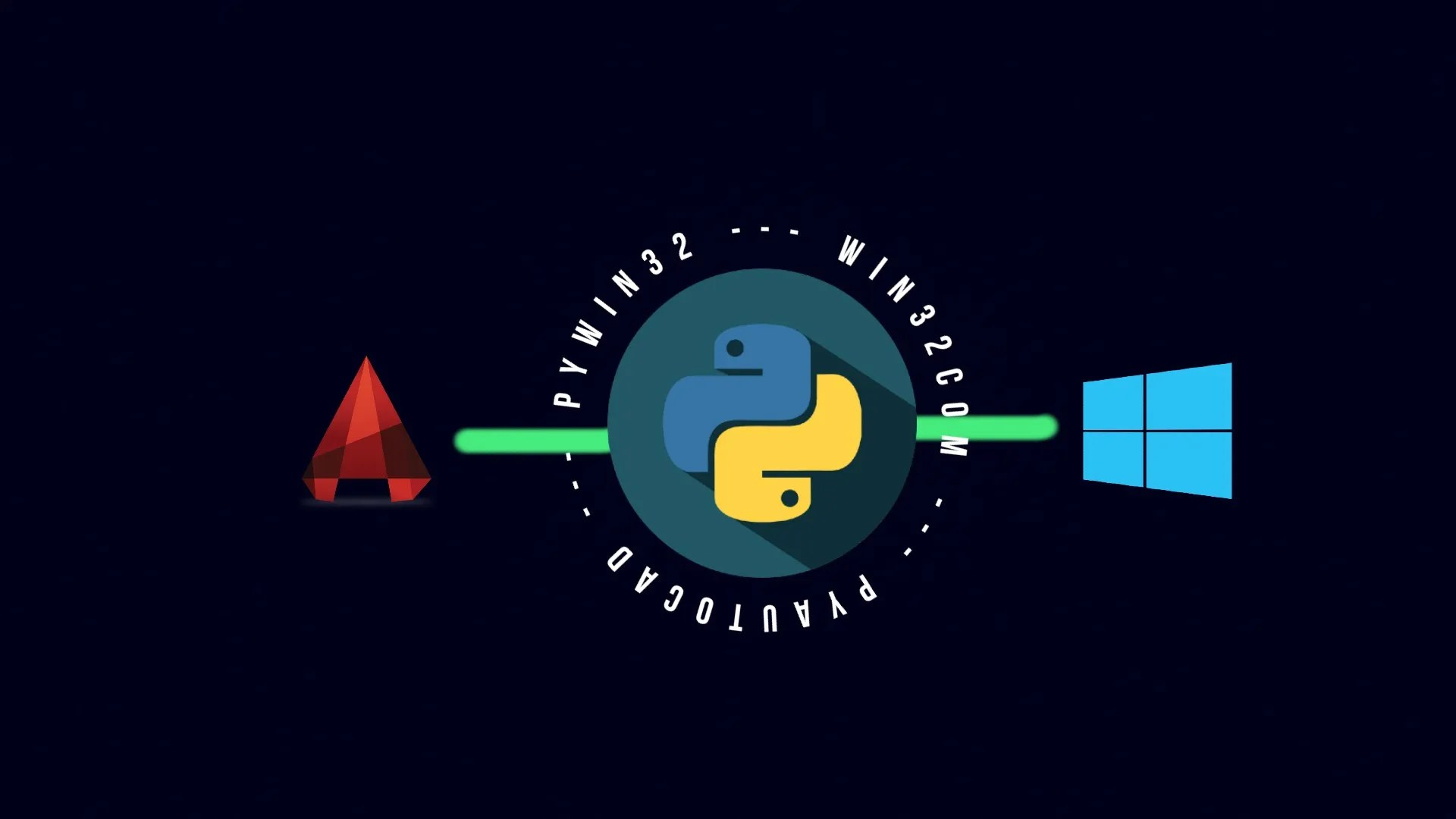In my previous post I discussed and demonstrated how to check for intersecting AutoCAD objects with pyautocad in Python. In this post I want to do something similar yet different: I want to show how you can extend AutoCAD objects so that they intersect. I want to do so in Python, using pyautocad. Here I provide a step-by-step guide for doing so.

## Understanding the EXTEND AutoCAD command in ActiveX

While working with AutoCAD most users frequently use the EXTEND command to extend different AutoCAD drawing objects. But ActiveX, the framework also used by pyautocad for scripting AutoCAD workflows, does not provide us with a method explicitly for extending objects to their maximum limits. That is, ActiveX does not provide a method or function that represents a direct equivalent to the AutoCAD EXTEND command.

In this case I refer to the maximum limit as being the process of extending objects up to a point where the first object intersects a second object. In AutoCAD there is a command for this, the EXTEND command.

Since ActiveX, and thus also pyautocad, does not provide a method explicitly for extending objects up to their maximum limits I myself need to implement a custom method for implementing this in Python.

As I now already explained that extending an AutoCAD object is related to the intersection of two objects and I also already demonstrated that the IntersectWith method can be used for detecting intersecting objects, I can now define the intersection point of two objects and extend objects based on this knowledge. From my earlier blog posts I know that I can change AutoCAD object properties in Python. I can do so by making use of the pyautocad module. Considering the same, I can extend AutoCAD objects by applying the IntersectWith method. I follow up with some code on this in a section further below. But first, I want to explain some method parameters.

## Extending objects based on the acExtendThisEntity parameter in IntersectWith pyautocad-method

As I discussed in my previous blog post regarding the IntersectWith method I have 4 conditions that I can control: acExtendNone, acExtendThisEntity, acExtendOtherEntity, and acExtendBoth.

From this I know that objects are already intersecting in the first conditions i.e. acExtendNone. So I will not be using this command to build my custom EXTEND AutoCAD command in Python.

Considering the second criteria, while working with the first object which we want to extend between the selected two, we can reuse and apply the code displayed below. By default, this code is built to extend the objects from the nearest possible end from the intersection point. Also, we have a choice to select at which point we want our first object to intersect the second one, in the case of multiple intersection points being feasible i.e. possible.

``````# condition 1: acExtendThisEntity
def extend(obj1, obj2):
if obj1.IntersectWith(obj2, 1) > (0, 0, 0):
intersection_points = split_tuple(obj1.IntersectWith(obj2, 1))
if len(intersection_points) == 0:
print("Condition 1 satisfies ", end="")
print(split_tuple(obj1.IntersectWith(obj2, 1)))
if math.dist(obj1.EndPoint, split_tuple(obj1.IntersectWith(obj2, 1))) < math.dist(obj1.StartPoint, split_tuple(obj1.IntersectWith(obj2, 1))):
obj1.EndPoint = APoint(intersection_points)
else:
obj1.StartPoint = APoint(intersection_points)
else:
print("Condition 1 satisfies ", end="")
print(split_tuple(obj1.IntersectWith(obj2, 1)))
end_point_index = int(input("There are more than one intersection points, please select the index value of specific intersection point: "))
if math.dist(obj1.EndPoint, APoint(intersection_points[end_point_index])) < math.dist(obj1.StartPoint, APoint(intersection_points[end_point_index])):
obj1.EndPoint = APoint(intersection_points[end_point_index])
else:
obj1.StartPoint = APoint(intersection_points[end_point_index])``````

## Extending objects based on acExtendOtherEntity parameter in IntersectWith method (pyautocad)

Now, for the other criteria i.e. acExtendOtherEntity, I can use the following code. It works the same way as that of the previous one. The only difference is that this works on extending the second object that I pass as a parameter.

``````# condition 2: acExtendOtherEntity
elif obj1.IntersectWith(obj2, 2) > (0, 0, 0):
intersection_points = split_tuple(obj1.IntersectWith(obj2, 2))
if len(intersection_points) == 0:
print("Condition 2 satisfies ", end="")
print(split_tuple(obj1.IntersectWith(obj2, 2)))
if math.dist(obj2.EndPoint, split_tuple(obj1.IntersectWith(obj2, 2))) < math.dist(obj1.StartPoint, split_tuple(obj1.IntersectWith(obj2, 2))):
obj2.EndPoint = APoint(intersection_points)
else:
obj2.StartPoint = APoint(intersection_points)
else:
print("Condition 2 satisfies ", end="")
print(split_tuple(obj1.IntersectWith(obj2, 2)))
end_point_index = int(input("There are more than one intersection points, please select the index value of specific intersection point: "))
if math.dist(obj2.EndPoint, APoint(intersection_points[end_point_index])) < math.dist(obj2.StartPoint, APoint(intersection_points[end_point_index])):
obj2.EndPoint = APoint(intersection_points[end_point_index])
else:
obj2.StartPoint = APoint(intersection_points[end_point_index])``````

## Extending object based on acExtendBoth parameter in IntersectWith method (pyautocad)

Finally, I can work for the last criterion which is acExtendBoth. In any case, this criterion will always fetch the nearest possible intersection point using the IntersectWith method itself. So, here I do not need to write some code to find the nearest possible point.

``````# condition 3: acExtendBoth
elif obj1.IntersectWith(obj2, 3) > (0, 0, 0):
intersection_points = obj1.IntersectWith(obj2, 3)
print("Condition 3 satisfies ", end="")
print(obj1.IntersectWith(obj2, 3))
obj1.EndPoint = APoint(intersection_points)
obj2.EndPoint = APoint(intersection_points)

# if objects are not intersecting each other.
else:
print("The objects does not intersect each other.")``````

## Implementing the extend method created using pyautocad

Now that I have got our code ready I can try to implement and test it against some objects.

``````c1 = acad.model.AddCircle(APoint(1000, 750), 200)

extend(c1, l1)

O/p:
Condition 2 satisfies [(1173.1931182546593, 850.0207168002088, 0.0), (1009.6731237835572, 550.2340602698549, 0.0)]
There are more than one intersection points, please select the index value of specific intersection point: 1``````

As I illustrated in Figure 1.1 and the code further above, the code chose to extend from its geometrical start point and not its geometrical endpoint. That means that the endpoint (1555, 1550, 0) is the same as before and that the line got extended from its start point (1255, 1000, 0) to (1009.6731237835572, 550.2340602698549, 0.0).